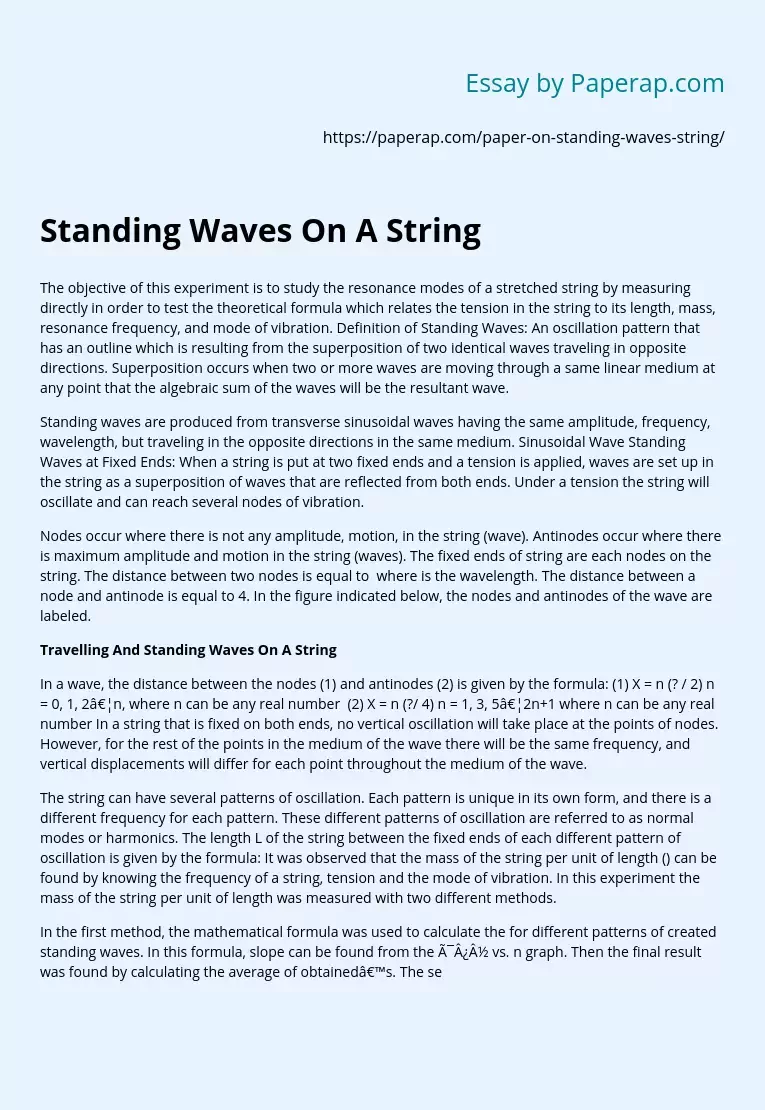# Running and Standing Waves on a String

The following sample essay talks about traveling and standing waves on a string. To read the introduction, body, and conclusion of the essay, scroll down.

The objective of this experiment is to study the resonance modes of a stretched string by measuring directly in order to test the theoretical formula which relates the tension in the string to its length, mass, resonance frequency, and mode of vibration. Definition of Standing Waves: An oscillation pattern that has an outline which is resulting from the superposition of two identical waves traveling in opposite directions.

Superposition occurs when two or more waves are moving through a same linear medium at any point that the algebraic sum of the waves will be the resultant wave.

Standing waves are produced from transverse sinusoidal waves having the same amplitude, frequency, wavelength, but traveling in the opposite directions in the same medium. Sinusoidal Wave Standing Waves at Fixed Ends: When a string is put at two fixed ends and a tension is applied, waves are set up in the string as a superposition of waves that are reflected from both ends.

Under a tension the string will oscillate and can reach several nodes of vibration.

Nodes occur where there is not any amplitude, motion, in the string (wave). Antinodes occur where there is maximum amplitude and motion in the string (waves). The fixed ends of string are each nodes on the string. The distance between two nodes is equal to  where is the wavelength. The distance between a node and antinode is equal to 4.

Get quality help nowWriter LylaVerified

Proficient in: Force5 (876)

“ Have been using her for a while and please believe when I tell you, she never fail. Thanks Writer Lyla you are indeed awesome ”+84 relevant experts are online

In the figure indicated below, the nodes and antinodes of the wave are labeled.

In a wave, the distance between the nodes (1) and antinodes (2) is given by the formula: (1) X = n (? / 2) n = 0, 1, 2…n, where n can be any real number  (2) X = n (?/ 4) n = 1, 3, 5…2n+1 where n can be any real number In a string that is fixed on both ends, no vertical oscillation will take place at the points of nodes. However, for the rest of the points in the medium of the wave there will be the same frequency, and vertical displacements will differ for each point throughout the medium of the wave.

The string can have several patterns of oscillation. Each pattern is unique in its own form, and there is a different frequency for each pattern. These different patterns of oscillation are referred to as normal modes or harmonics. The length L of the string between the fixed ends of each different pattern of oscillation is given by the formula: It was observed that the mass of the string per unit of length () can be found by knowing the frequency of a string, tension and the mode of vibration. In this experiment the mass of the string per unit of length was measured with two different methods.

In the first method, the mathematical formula was used to calculate the for different patterns of created standing waves. In this formula, slope can be found from the ï¿½ vs. n graph. Then the final result was found by calculating the average of obtained’s. The second method was measuring the directly by using the formula , where the mass of the string was determined by using the electrical balance. The average that was calculated in the first method was (4.84 0.05) 10-4 Kg/m, and The value of by using the direct measurement was 5.1010-4 Kg/m 1.03  10-6 Kg/m. These two results are close to reach other, but the result by using the second way is more accurate because of the fact that in the second method an electrical balance was used to measure the weight of the string which is relatively more accurate.

Since the mass of the string per unit of length values obtained through the both methods had minimum differences, this experiment was relatively a success. When finding resonance frequencies it was also known that the 2nd mode of vibration should be double the fundamental frequency. For example3 = 1 and so on. Although the values obtained for higher modes of vibration were close to being multiples of one another, they were not exact.

The causes of why these errors have occurred are due to a number of reasons. The air resistance could have affected the standing wave of the string which consequently affects the frequency of the wave. When the string reaches higher modes of vibration becomes more difficult to sense where the nodes are which will impact the frequency. There also could have been some errors in weighing and measuring the length of the string. To reduce the errors of this experiment it could have been performed several times.